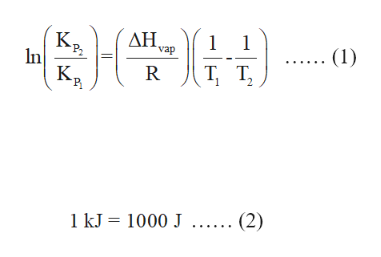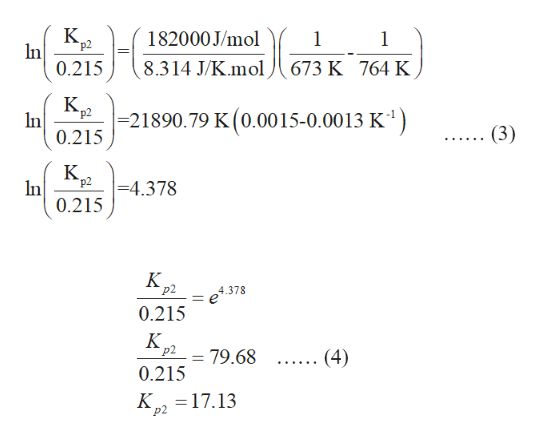# The equilibrium constant, Kp, for the following reaction is 0.215 at 673 K.NH4I(s) NH3(g) + HI(g)If ΔH° for this reaction is 182 kJ, what is the value of Kp at 764 K?Kp = ______

Question
The equilibrium constant, Kp, for the following reaction is 0.215 at 673 K.

NH4I(s) NH3(g) + HI(g)

If ΔH° for this reaction is 182 kJ, what is the value of Kp at 764 K?

Kp = ______

check_circleExpert Solution
Step 1

The expression for van’t Hoff equation is expressed in equation (1) in which KP1 and KP2 are equilibrium constants, T1 and T2 are two different temperatures, ∆H is the enthalpy for the reaction and R is the gas constant.

The relation between joule and kilojoule is labelled in equation (2).help_outlineImage TranscriptioncloseК, In K К, P ΔΗ vap 1 1 (1) Т, т, R T, T, 2 1 kJ 1000 J..... (2) fullscreen
Step 2

Convert 182 kJ into joules by using the equation (2).

182 kJ = 182 ×1000J

= 182000 J

Step 3

Use the equation (2) and substitute 0.215 for KP1, 673 K for T1, 764 K for T2 , 8.314 J/K.mol for R and 182000 J/mol for ∆H○ as shown below i...help_outlineImage TranscriptioncloseK. р2 In 182000J/mol 1 1 8.314 J/K.mol 0.215 673 K 764 K К, In 21890.79 K (0.0015-0.0013 K) 0.215 р2 (3) К. р2 -4.378 In 0.215 К, р2 4.378 0.215 К. P2 .... (4) 79.68 0.215 K 17.13 р2 fullscreen

### Want to see the full answer?

See Solution

#### Want to see this answer and more?

Solutions are written by subject experts who are available 24/7. Questions are typically answered within 1 hour*

See Solution
*Response times may vary by subject and question
Tagged in

### Chemistry